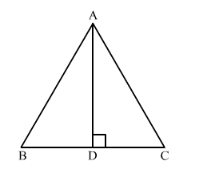# In a ∆ABC, AB = BC = CA = 2a and AD ⊥ BC. Prove that

Question:

In a $\triangle A B C, A B=B C=C A=2 a$ and $A D \perp B C$. Prove that

(i) $\mathrm{AD}=a \sqrt{3}$

(ii) Area $(\Delta A B C)=\sqrt{3} a^{2}$

Solution:In $\triangle A D B$ and $\triangle A D C$

$\angle B=\angle C \quad\left(60^{\circ}\right.$ each $)$

$A D=A D$

$\angle A D B=\angle A D C \quad\left(90^{\circ}\right.$ each $)$

$\triangle A D B \cong \triangle A D C \quad$ (AAS congruence theorem)

$\therefore B D=D C$

$\therefore B C=2 B D$

But $B C=2 a$ therefore, we get,

$2 a=2 B D$....(1)

Now we will divide both sides of the equation (1) by 2, we get,

$\therefore B D=a$

Now we will use Pythagoras theorem in right angled triangle ADB.

$A B^{2}=A D^{2}+B D^{2}$

Now we will substitute the values of AB and BD we get,

$(2 a)^{2}=A D^{2}+a^{2}$

$4 a^{2}=A D^{2}+a^{2}$

$3 a^{2}=A D^{2}$

$\therefore A D=\sqrt{3} a$

Therefore, $A D=\sqrt{3} a$.

We have given an equilateral triangle and we know that the area of the equilateral triangle is $\frac{\sqrt{3}}{4} \times$ side $^{2}$

Here, side is 2a

$\therefore A(\Delta A B C)=\frac{\sqrt{3}}{4} \times(2 a)^{2}$

$\therefore A(\triangle A B C)=\frac{\sqrt{3}}{4} \times 4 a^{2}$

$A(\triangle A B C)=\sqrt{3} a^{2}$

Therefore, $A(\triangle A B C)=\sqrt{3} a^{2}$.